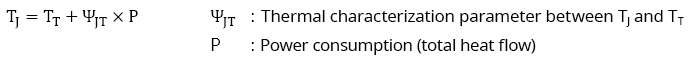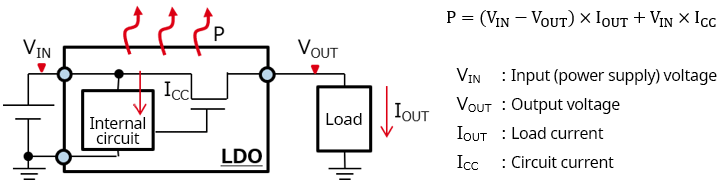Technical Information Site of Power Supply Design

2022.03.09 Thermal Design

# Estimating TJ: Basic Calculation Equations

Thermal Design of Semiconductor Components in Electronic Equipment

Up till now, to facilitate understanding of thermal resistance data, we have explained how θJA and ΨJT are used, or can be used, in calculations to estimate TJ, and have discussed the characteristics of ΨJT and the usefulness of θJA and ΨJT in estimating TJ. From here, we will present examples of calculations to estimate TJ using thermal resistance data.

## Basic Calculation Equations Used in Estimation of TJ

We begin with the basic calculation equations used in estimation of TJ. TJ can be estimated using either of the following two equations.

① Using the ambient temperature TA② Using the temperature TT at the center of the top surface of the IC package in the state of actual useWhichever equation is used, the power consumption P is essentially the product of the power supply voltage and the power supply current for the IC, but the IC functions (whether it is a power supply IC, an op-amp, etc.), the output load current, and other factors must be considered. In all cases, however, the power consumption is determined from the current and voltage consumed by the IC. Shown below is an example of a linear regulator.This is the simplest example; the power consumption of the IC itself is VIN×ICC, the power consumption of the load is the input/output difference (VIN-VOUT) ×IOUT, and the sum of the two is the power consumption for the IC as a whole.

From the next article, examples are used in actual determination of TJ.

#### Key Points:

・There are two methods for estimating TJ: using TA and θJA, or using TT and ΨJT.

・In both calculations, the IC power consumption P is necessary.